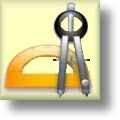#Geometry Worksheets

## Volume Worksheets

Here is a graphic preview for all of the Surface Area & Volume Worksheets. You can select different variables to customize these Surface Area & Volume Worksheets for your needs. The Surface Area & Volume Worksheets are randomly created and will never repeat so you have an endless supply of quality Surface Area & Volume Worksheets to use in the classroom or at home. We have identifying solid figures, surface area & volume of prisms and cylinders, surface area & volume of pyramids and cones, and surface area & volume of spheres for your use. Our Surface Area & Volume Worksheets are free to download, easy to use, and very flexible.

These Surface Area & Volume Worksheets are a great resource for children in 5th, 6th Grade, 7th Grade, and 8th Grade.

## Detailed Description for All Volume Worksheets

Surface Area and Volume Handout
These Surface Area and Volume Handouts has useful definitions, facts, and formulas for cubes, rectangular prisms, general prisms, cylinders, pyramids, cones, and spheres. These worksheets are a great resources for the 5th, 6th Grade, 7th Grade, 8th Grade, 9th Grade, and 10th Grade.

Identify Solid Figures Worksheets
These Surface Area and Volume Worksheets will produce twelve problems for identifying different types of solid figures. These worksheets are a great resources for the 5th, 6th Grade, 7th Grade, 8th Grade, 9th Grade, and 10th Grade.

Prisms and Cylinders Surface Area Worksheets
These Surface Area and Volume Worksheets will produce problems for calculating surface area for prisms and cylinders. You may select the units of measurement for each problem. These worksheets are a great resources for the 5th, 6th Grade, 7th Grade, 8th Grade, 9th Grade, and 10th Grade.

Prisms and Cylinders Volume Worksheets
These Surface Area and Volume Worksheets will produce problems for calculating volume for prisms and cylinders. You may select the units of measurement for each problem. These worksheets are a great resources for the 5th, 6th Grade, 7th Grade, 8th Grade, 9th Grade, and 10th Grade.

Prisms and Pyramids Surface Area Worksheets
These Surface Area and Volume Worksheets will produce problems for calculating surface area for prisms and pyramids. You may select the units of measurement for each problem. These worksheets are a great resources for the 5th, 6th Grade, 7th Grade, 8th Grade, 9th Grade, and 10th Grade.

Prisms and Pyramids Volume Worksheets
These Surface Area and Volume Worksheets will produce problems for calculating volume for prisms and pyramids. You may select the units of measurement for each problem. These worksheets are a great resources for the 5th, 6th Grade, 7th Grade, 8th Grade, 9th Grade, and 10th Grade.

Pyramids and Cones Surface Area Worksheets
These Surface Area and Volume Worksheets will produce problems for calculating surface area for pyramids and cones. You may select the units of measurement for each problem. These worksheets are a great resources for the 5th, 6th Grade, 7th Grade, 8th Grade, 9th Grade, and 10th Grade.

Pyramids and Cones Volume Worksheets
These Surface Area and Volume Worksheets will produce problems for calculating volume for pyramids and cones. You may select the units of measurement for each problem. These worksheets are a great resources for the 5th, 6th Grade, 7th Grade, 8th Grade, 9th Grade, and 10th Grade.

Cylinders and Cones Surface Area Worksheets
These Surface Area and Volume Worksheets will produce problems for calculating surface area for cylinders and cones. You may select the units of measurement for each problem. These worksheets are a great resources for the 5th, 6th Grade, 7th Grade, 8th Grade, 9th Grade, and 10th Grade.

Cylinders and Cones Volume Worksheets
These Surface Area and Volume Worksheets will produce problems for calculating volume for cylinders and cones. You may select the units of measurement for each problem. These worksheets are a great resources for the 5th, 6th Grade, 7th Grade, 8th Grade, 9th Grade, and 10th Grade.

Prisms, Pyramids, Cylinders and Cones Surface Area Worksheets
These Surface Area and Volume Worksheets will produce problems for calculating surface area for prisms, pyramids, cylinders and cones. You may select the units of measurement for each problem. These worksheets are a great resources for the 5th, 6th Grade, 7th Grade, 8th Grade, 9th Grade, and 10th Grade.

Prisms, Pyramids, Cylinders and Cones Volume Worksheets
These Surface Area and Volume Worksheets will produce problems for calculating volume for prisms, pyramids, cylinders and cones. You may select the units of measurement for each problem. These worksheets are a great resources for the 5th, 6th Grade, 7th Grade, 8th Grade, 9th Grade, and 10th Grade.

Spheres Surface Area and Volume Worksheets
These Surface Area and Volume Worksheets will produce problems for calculating surface area and volume for spheres. You may select the units of measurement for each problem. These worksheets are a great resources for the 5th, 6th Grade, 7th Grade, 8th Grade, 9th Grade, and 10th Grade.

Nets of Solids Worksheets
These Surface Area and Volume Worksheets will produce problems for identifying and sketching nets of solids. You can select the types of solids to work with. These worksheets are a great resources for the 5th, 6th Grade, 7th Grade, 8th Grade, 9th Grade, and 10th Grade.

Similar Solids Worksheets
These Surface Area and Volume Worksheets will produce problems for identifying and sketching similar solids. You can select the types of solids to work with. These worksheets are a great resources for the 5th, 6th Grade, 7th Grade, 8th Grade, 9th Grade, and 10th Grade.